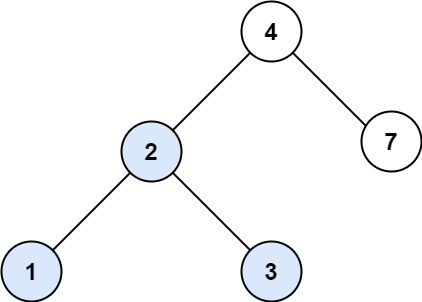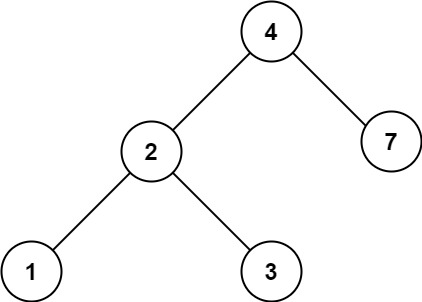# GeetCode Hub

You are given the `root` of a binary search tree (BST) and an integer `val`.

Find the node in the BST that the node's value equals `val` and return the subtree rooted with that node. If such a node does not exist, return `null`.

Example 1:```Input: root = [4,2,7,1,3], val = 2
Output: [2,1,3]
```

Example 2:```Input: root = [4,2,7,1,3], val = 5
Output: []
```

Constraints:

• The number of nodes in the tree is in the range `[1, 5000]`.
• `1 <= Node.val <= 107`
• `root` is a binary search tree.
• `1 <= val <= 107`

/** * Definition for a binary tree node. * public class TreeNode { * int val; * TreeNode left; * TreeNode right; * TreeNode() {} * TreeNode(int val) { this.val = val; } * TreeNode(int val, TreeNode left, TreeNode right) { * this.val = val; * this.left = left; * this.right = right; * } * } */ class Solution { public TreeNode searchBST(TreeNode root, int val) { } }